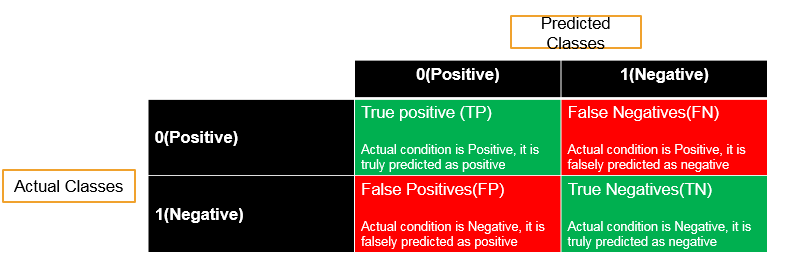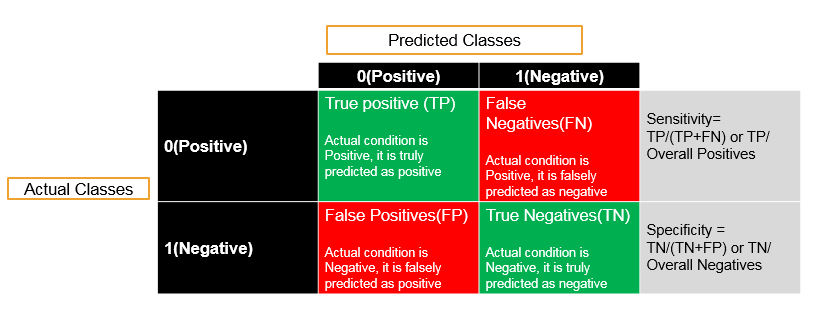• No products in the cart.

# 204.4.1 Model Section and Cross Validation

##### Starting off with introduction to Model Validation

Building a model is not that difficult. However, tuning the model and checking if the model is working as we have built it to is a different game.

In this series, we will be covering methods and matrices to validate the model and find an optimum model for our requirement.

## Model Validation Metrics

### Model Validation

• Checking how good is our model
• It is very important to report the accuracy of the model along with the final model
• The model validation in regression is done through R square and Adj R-Square
• Logistic Regression, Decision tree and other classification techniques have very similar validation measures.
• Till now we have seen confusion matrix and accuracy. There are many more validation and model accuracy metrics for classification models.

### Classification-Validation measures

• Confusion matrix, Specificity, Sensitivity
• ROC, AUC
• KS, Gini
• Concordance and discordance
• Chi-Square, Hosmer and Lemeshow Goodness-of-Fit Test
• Lift curve

All of them are measuring the model accuracy only. Some metrics work really well for certain class of problems. Confusion matrix, ROC and AUC will be sufficient for most of the business problems.

### Sensitivity and Specificity

Sensitivity and Specificity are derived from confusion matrix.• Accuracy=(TP+TN)/(TP+FP+FN+TN)
• Misclassification Rate=(FP+FN)/(TP+FP+FN+TN)
• Sensitivity : Percentage of positives that are successfully classified as positive
• Specificity : Percentage of negatives that are successfully classified as negativesIn next session session we will find ways to calculate sensitivity and specificity in Python.

Link to the next post : https://statinfer.com/204-4-2-calculating-sensitivity-and-specificity-in-python/

### 0 responses on "204.4.1 Model Section and Cross Validation"

Statinfer Software Solutions LLP

Software Technology Parks of India,
NH16, Krishna Nagar, Benz Circle,